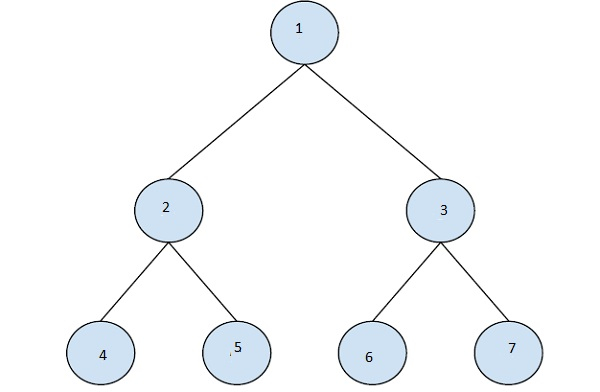# Find n-th node of inorder traversal in C++

In this problem, we are given a binary tree and an integer N. The task is to find the n-th node in inorder traversal of a Binary Tree.

A binary tree has a special condition that each node can have a maximum of two children.

Traversal is a process to visit all the nodes of a tree and may print their values too.

Let’s take an example to understand the problem,

## Input

N = 6## Output

3

## Explanation

inorder traversal of tree : 4, 2, 5, 1, 6, 3, 7

## Solution Approach

The idea is to use the in-order traversal of the binary tree which is done by using recursive calls. In each call we will call preOrder() for left subtree first and traverse the root node then call preOrder(). During this traversal, we will count the number of nodes and print the node for which the count is N.

Program to illustrate the working of our solution,

## Example

Live Demo

#include <iostream>
using namespace std;
struct Node {
int data;
Node *left, *right;
};
struct Node* createNode(int item){
Node* temp = new Node;
temp->data = item;
temp->left = NULL;
temp->right = NULL;
return temp;
}
void findInOrderTraversalRec(struct Node* node, int N){
static int count = 0;
if (node == NULL)
return;
if (count <= N) {
findInOrderTraversalRec(node->left, N);
count++;
if (count == N)
cout<<node->data;
findInOrderTraversalRec(node->right, N);
}
}
int main() {
struct Node* root = createNode(1);
root->left = createNode(2);
root->right = createNode(3);
root->left->left = createNode(4);
root->left->right = createNode(5);
root->right->left = createNode(6);
root->right->right = createNode(7);
int N = 6;
cout<<N<<"th node in inorder traversal is ";
findInOrderTraversalRec(root, N);
return 0;
}

## Output

6th node in inorder traversal is 3

Updated on: 13-Mar-2021

208 Views